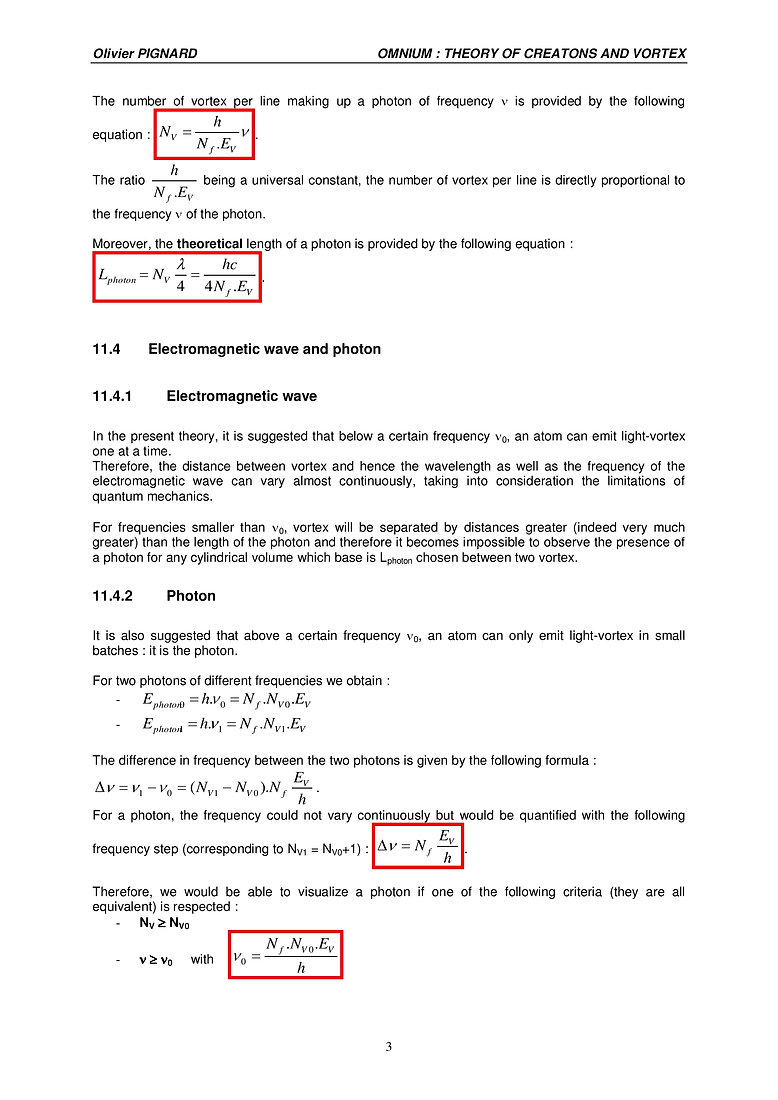## Chapter 11 - Presentation of lines of light-vortex / photon

Download the complete chapter## 11.1 Line of light-vortex

The line of light-vortex is what makes up a photon. Vortex have an axis which is perpendicular to the direction of propagation, as shown by the figure below.

In a uniform field of creatons, light-vortex have a rectilinear movement at the speed of light “in the vacuum”.

Each vortex is a wave and the line of vortex looks like a group of waves propagating on the surface of the ocean.

11.2 Model of the photon

In 1905, Albert Einstein showed that light is not distributed homogeneously in space but that its energy is quantified. This led to the introduction of the photon, the smallest quantum of energy for light, particle of energy .

What my theory suggests is that the photon itself is not an elementary particle but is made up of vortex. Subsequently the energy of the photon is not uniformly distributed in the space occupied by the photon but is localized, condensed in the form of vortex.

It is considered that the photon is constituted by Nf lines of vortex, each possessing NV vortex.

The number of lines Nf is a constant integer regardless of the frequency of the photon.

The following diagram represents a photon which in this example possesses Nf = 9 lines of Nv = 7 vortex each.

11.3 Energy and length of a photon

Albert Einstein gave the energy of a photon in 1905, extending Max Planck's work, with the well-known formula : .

Therefore the energy of a photon increases linearly with its frequency.

This is clarified in the theory I am putting forward by the fact that the number of vortex that make up a photon increases also with the frequency.

In our model, the length of the photon, the dimension along the direction of propagation, is considered to be constant.

The average distance between vortex in a line being l/4, the length of a photon is given by the formula    where NV represents the number of vortex in a line which makes up the photon.

In the present theory, the vortex and the photon possess a mass (very weak but not null).

The energy of a ”light-vortex” is given by the formula : .

It is believed that the energy EV of a vortex is a universal constant on the same basis as the speed of light or Planck's constant.

The energy of a photon is given by the following formula :

From the preceding equations we can infer the fundamental formula giving the frequency :

The number of vortex per line making up a photon of frequency n is provided by the following equation : .

The ratio  being a universal constant, the number of vortex per line is directly proportional to the frequency n of the photon.

Moreover, the theoretical length of a photon is provided by the following equation :

11.4 Electromagnetic wave and photon

11.4.1 Electromagnetic wave

In the present theory, it is suggested that below a certain frequency n0, an atom can emit light-vortex one at a time.

Therefore, the distance between vortex and hence the wavelength as well as the frequency of the electromagnetic wave can vary almost continuously, taking into consideration the limitations of quantum mechanics.

For frequencies smaller than n0, vortex will be separated by distances greater (indeed very much greater) than the length of the photon and therefore it becomes impossible to observe the presence of a photon for any cylindrical volume which base is Lphoton chosen between two vortex.

11.4.2 Photon

It is also suggested that above a certain frequency n0, an atom can only emit light-vortex in small batches : it is the photon.

For two photons of different frequencies we obtain :

The difference in frequency between the two photons is given by the following formula :

For a photon, the frequency could not vary continuously but would be quantified with the following frequency step (corresponding to NV1 = NV0+1) : .

Therefore, we would be able to visualize a photon if one of the following criteria (they are all equivalent) is respected :

It is therefore possible to write   as well as .

On the other hand, for a wavelength l bigger than l0, only an electromagnetic wave will be present.

It is no longer possible to speak of a photon.

For example for n = 3 MHz, we obtain l = 100 meters. The vortex are separated by a distance of 25 meters and hence a particle between two consecutive vortex cannot be located.

It is important to understand that, in spite of this frequency n0 delineating the domain of electromagnetic waves and that of photons, it is the same fundamental physical phenomena which is at work in both cases : the propagation of light-vortex at the speed of light IRRESPECTIVE OF THE FREQUENCY n covering the totality of the frequency spectrum.